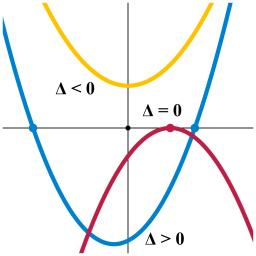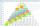# Sequence

In the arithmetic sequence is given:

Sn=2304, d=2, an=95

Calculate a1 and n.

a =  1
n =  48

### Step-by-step explanation:

Our quadratic equation calculator calculates it.

$n={n}_{1}=48=48$Did you find an error or inaccuracy? Feel free to write us. Thank you!Tips to related online calculators
Looking for help with calculating roots of a quadratic equation?
Do you have a system of equations and looking for calculator system of linear equations?

## Related math problems and questions:

• AP 6Calculate the first five items of an arithmetic sequence if it is given: a2 – a3 + a5 = 20 a1 + a6 = 38
• Geometric sequence 5About members of geometric sequence we know: ? ? Calculate a1 (first member) and q (common ratio or q-coefficient)
• Geometric sequenceIn the geometric sequence is a4 = 20 a9 = -160. Calculate the first member a1 and quotient q.
• SequenceIn the arithmetic sequence is a1=-1, d=4. Which member is equal to the number 203?
• Geometric sequence 3In geometric sequence is a8 = 312500; a11= 39062500; sn=1953124. Calculate the first item a1, quotient q, and n - number of members by their sum s_n.
• SequenceWrite the first 6 members of this sequence: a1 = 5 a2 = 7 an+2 = an+1 +2 an
• SequenceWrite the first 7 members of an arithmetic sequence: a1=-3, d=6.
• Finite arithmetic sequenceHow many numbers should be inserted between the numbers 1 and 25 so that all numbers create a finite arithmetic sequence and that the sum of all members of this group is 117?
• The sumThe sum of the squares of two immediately following natural numbers is 1201. Find these numbers.
• Sequences AP + GPThe three numbers that make up the arithmetic sequence have the sum of 30. If we subtract from the first 5, from the second 4 and keep the third, we get the geometric sequence. Find AP and GP members.
• The sidesThe sides of a right triangle form an arithmetic sequence. The hypotenuse is 24 cm long. Determine the remaining sides of the triangle.
• Rectangular triangleThe lengths of the rectangular triangle sides with a longer leg of 12 cm form an arithmetic sequence. What is the area of the triangle?
• Sequence 2Write the first 5 members of an arithmetic sequence a11=-14, d=-1
• Tenth memberCalculate the tenth member of geometric sequence when given: a1=1/2 and q=2
• Two-digit numberDigit sum of thinking two-digit natural number is 11. When it exchanging a sequence of digits, given a number which is 27 less than the thinking number. Find out which number I think.
• Arithmetic progressionIn some AP applies: 5a2 + 7a5 = 90 s3 = 12 Find the first member a =? and difference d = ?
• Geometric seqFind the third member of geometric progression if a1 + a2 = 36 and a1 + a3 = 90. Calculate its quotient.